# ML Aggarwal Solutions Class 10 Maths Chapter 11 Section Formula

1. Find the co-ordinates of the mid-point of the line segments joining the following pairs of points:
(i) (2,– 3),( – 6,7)
(ii) (5,– 11),(4,3)
(iii) (a + 3,5b),(2a – 1,3b + 4)
Solution:
We have given that:
Co-ordinates of midpoint of line joining the points (x1,y1) and (x2,y2) = {(x1+x2)/2 ,(y1+y2)/2}
(i) Co-ordinates of midpoint of line joining the points (2,-3) and (-6,7) = {(2+-6)/2,(-3+7)/2}
= (-4/2,4/2)
= (-2,2)
Hence the co-ordinates of midpoint of line joining the points (2,-3) and (-6,7) is (-2,2).
(ii) Co-ordinates of midpoint of line joining the points (x1,y1) and (x2,y2) = {(x1+x2)/2 ,(y1+y2)/2}
Co-ordinates of midpoint of line joining the points (5,-11) and (4,3) = {(5+4)/2,(-11+3)/2}
= (9/2,-8/2)
= (9/2,-4)
Hence the co-ordinates of midpoint of line joining the points (5,-11) and (4,3) is (9/2,-4).
(iii) Co-ordinates of midpoint of line joining the points (x1,y1) and (x2,y2) = {(x1+x2)/2 ,(y1+y2)/2}
Co-ordinates of midpoint of line joining the points (a+3,5b) and (2a-1,3b+4) = {(a+3+2a-1)/2,(5b+3b+4)/2}
= {(3a+2)/2,(8b+4)/2}
= {(3a+2)/2,(4b+2)}
Hence the co-ordinates of midpoint of line joining the points (a+3,5b) and (2a-1,3b+4) are {(3a+2)/2,(4b+2)}.

2. The co-ordinates of two points A and B are ( – 3,3) and (12,– 7) respectively. P is a point on the line segment AB such that AP∶ PB = 2∶ 3. Find the co-ordinates of P.
Solution:
We have given that:
Let the co-ordinates of P(x,y) divides AB in the ratio m:n.
A(-3,3) and B(12,-7) are the given points.
Given m:n = 2:3
x1  = -3 ,y1 = 3 , x2  = 12 ,y2  = -7 ,m = 2 and n = 3
By Section formula x = (mx2+nx1)/(m+n)
x = (2×12+3×-3)/(2+3)
x = (24-9)/5
x = 15/5
x = 3
By Section formula y = (my2+ny1)/(m+n)
y = (2×-7+3×3)/5
y = (-14+9)/5
y = -5/5
y = -1
Hence, the given values y = -1 are not roots of the equation.
Hence the co-ordinate of point P are (3,-1).

3. P divides the distance between A ( – 2,1) and B (1,4) in the ratio of 2∶ 1. Calculate the co-ordinates of the point P.
Solution:
We have given that:
Let the co-ordinates of P(x,y) divides AB in the ratio m:n.
A(-2,1) and B(1,4) are the given points.
Given m:n = 2:1
x1  = -2 ,y1  = 1 ,x = 1 ,y2= 4 ,m = 2 and n = 1
By Section formula x = (mx2+nx1)/(m+n)
x = (2×1+1×-2)/(2+1)
x = (2-2)/3
x = 0/3
x = 0
By Section formula y = (my2+ny1)/(m+n)
y = (2×4+1×1)/(2+1)
y = (8+1)/3
y = 9/3
y = 3
Hence, the given values y = 3are not roots of the equation.
Hence the co-ordinate of point P are (0,3).

4. (i) Find the co-ordinates of the points of trisection of the line segment joining the point (3,– 3) and (6,9).
(ii) The line segment joining the points (3,– 4) and (1,2) is trisected at the points P and Q. If the coordinates of P and Q are (p,– 2) and (5/3,q) respectively, find the values of p and q.
Solution:
We have given that: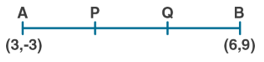Let P and Q be the points of trisection of AB
i.e., AP = PQ = QB
Given A(3,-3) and B(6,9)
x1   = 3, y1= -3,  x2  = 6, y2 = 9
P(x,y) divides AB internally in the ratio 1∶ 2.
m:n = 1:2
By applying the section formula, the coordinates of P are as follows.
By Section formula x = (mx2+nx1)/(m+n)
x = (1×6+2×3)/(1+2)
x = (6+6)/3
x = 12/3
x = 4
By Section formula y = (my2+ny1)/(m+n)
y = (1×9+2×-3)/(2+1)
y = (9-6)/3
y = 3/3
y = 1
Hence the co-ordinate of point P are (4,1).
Now, Q also divides AB internally in the ratio 2∶ 1.
m:n = 2:1
By applying the section formula, the coordinates of P are as follows.

x = (2×6+1×3)/(1+2)
x = (12+3)/3
x = 15/3
x = 5
By Section formula y = (my2+ny1)/(m+n)
y = (2×9+1×-3)/(2+1)
y = (18-3)/3
y = 15/3
y = 5
Hence, the given values y = 5 are not roots of the equation.
Hence the co-ordinate of point Q are (5,5).

(ii) Let P(p,-2) and Q(5/3,q) be the points of trisection of ABi.e., AP = PQ = QB
Given A(3,-4) and B(1,2)
x1   = 3,y1  = -4, x2  = 1, y2   = 2
P(p,-2) divides AB internally in the ratio 1∶ 2.
By Section formula x = (mx2+nx1)/(m+n)
p = (1×1+2×3)/(1+2)
p = (1+6)/3
p = 7/3
Now, Q also divides AB internally in the ratio 2∶ 1.
m:n = 2:1
Q(5/3,q) divides AB internally in the ratio 2∶ 1.
By Section formula y = (my2+ny1)/(m+n)
q = (2×2+1×-4)/(2+1)
q = (4-4)/3
q = 0/3
q = 0
Hence, the given values q = 0 are not roots of the equation.
Hence the value of p and q are 7/3 and 0 respectively.

5. (i) The line segment joining the points A (3,2) and B (5,1) is divided at the point P in the ratio 1∶ 2 and it lies on the line 3x – 18y + k = 0. Find the value of k.
(ii) A point P divides the line segment joining the points A (3,– 5) and B ( – 4,8) such that AP/PB = k/1  If P lies on the line x + y = 0, then find the value of k.
Solution:
We have given that:
(i) Let the co-ordinates of P(x,y) divides AB in the ratio m:n.
A(3,2) and B(5,1) are the given points.
Given m:n = 1:2
x1  = 3 ,y1  = 2 , x2  = 5 , y2 = 1 ,m = 1 and n = 2
By Section formula x = (mx2+nx1)/(m+n)
x = (1×5+2×3)/(1+2)
x = (5+6)/3
x = 11/3
By Section formula y = (my2+ny1)/(m+n)
y = (1×1+2×2)/(1+2)
y = (1+4)/3
y = 5/3
Given P lies on the line 3x-18y+k = 0
Substitute x and y in above equation
3×(11/3)-18×(5/3)+k = 0
11-30+k = 0
-19+k = 0
k = 19
Hence, the given values k = 19 are not roots of the equation.
Hence the value of k is 19.
(ii) Let the co-ordinates of P(x,y) divides AB in the ratio m:n.
A(3,-5) and B(-4,8) are the given points.
Given AP/PB = k/1
m:n = k:1
x1   = 3 , y1  = -5 , x2  = -4 ,y2  = 8 ,m = k and n = 1
By Section formula x = (mx2+nx1)/(m+n)
x = (k×-4+1×3)/(k+1)
x = (-4k+3)/(k+1)
x = (-4k+3)/(k+1)
By Section formula y = (my2+ny1)/(m+n)
y = (k×8+1×-5)/(k+1)
y = (-4k+3)/(k+1)
Co-ordinate of P is ((-4k+3)/(k+1),(8k-5)/(k+1))
Given P lies on line x+y = 0
Substitute value of x and y in above equation
(-4k+3)/(k+1) + (8k-5)/(k+1) = 0
(-4k+3) + (8k-5) = 0
4k-2 = 0
4k = 2
k = 2/4 = ½
Hence, the given values k = 2/4 = ½ are not roots of the equation.
Hence the value of k is ½ .

6. Find the coordinates of the point which is three-fourth of the way from A (3,1) to B ( – 2,5).
Solution:
We have given that:Let P be the point which is three-fourth of the way from A(3,1) to B(-2,5).
AP/AB = 3/ 4
AB = AP+PB
AP/AB = AP/(AP+PB) = 3/4
4AP = 3AP+3PB
4AP-3AP = 3PB
AP = 3PB
AP/PB = 3/1
The ratio m:n = 3:1
x1 = 3 ,y= 1 , x2 = -2,y2 = 5
By Section formula x = (mx2+nx1)/(m+n)
x = (3×-2+1×3)/(3+1)
x = (-6+3)/4
x = -3/4
By Section formula y = (my2+ny1)/(m+n)
y = (3×5+1×1)/(3+1)
y = (15+1)/4
y = 16/4
y = 4
Hence, the given values y=4 are not roots of the equation.
Hence the co-ordinates of P are (-3/4,4).

7. Point P (3,– 5) is reflected to P’ in the x- axis. Also P on reflection in the y-axis is mapped as P”.
(i) Find the co-ordinates of P’ and P”.
(ii) Compute the distance P’ P”.
(iii) Find the middle point of the line segment P’ P”.
(iv) On which co-ordinate axis does the middle point of the line segment P P” lie ?
Solution:
We have given that:
(i) The image of P(3,-5) when reflected in X-axis will be (3,5).
When you reflect a point across the X-axis, the x-coordinate remains the same,
but the y-coordinate is transformed into its opposite (its sign is changed).
Co-ordinates of P’ = (3,5)
Image of P(3,-5) when reflected in Y axis will be (-3,-5).
When you reflect a point across the Y-axis, the y-coordinate remains the same,
but the x-coordinate is transformed into its opposite (its sign is changed)
Co-ordinates of P’’ = (-3,-5)
(ii)Let P’(x1,y1) and P’’(x2  ,y2) be the given points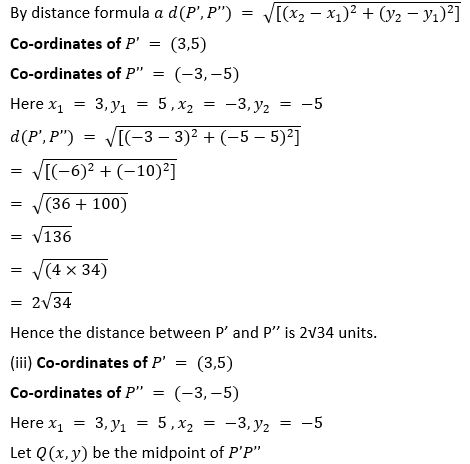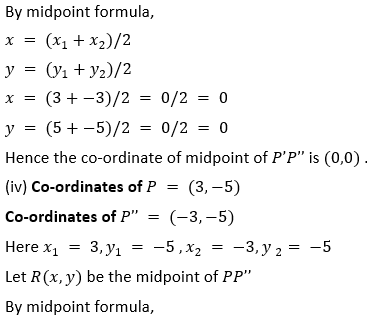x = (x1+x2)/2
y = (y1+y2)/2
x = (3+-3)/2 = 0/2 = 0
y = (-5+-5)/2 = -10/2 = -5
So the co-ordinate of midpoint of PP’’ is (0,-5) .
Here x co-ordinate is zero.
Hence the point lies on Y-axis.

8. Use graph paper for this question. Take 1 cm = 1 unit on both axes. Plot the points A(3,0) and B(0,4).
(i) Write down the co-ordinates of A1, the reflection of A in the y-axis.
(ii) Write down the co-ordinates of B1, the reflection of B in the x-axis.
(iii) Assign the special name to the quadrilateral ABA1B1.
(iv) If C is the midpoint is AB. Write down the co-ordinates of the point C1, the reflection of C in the origin.
(v) Assign the special name to quadrilateral ABC1B1.
Solution:
We have given that: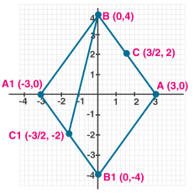(i) Co-ordinates of point A are (3,0).
When you reflect a point across the Y-axis, the y-coordinate remains the same,
but the x-coordinate is transformed into its opposite (its sign is changed)
Hence the reflection of A in the Y axis is (-3,0).
(ii) Co-ordinates of point B are (0,4).
When you reflect a point across the X-axis, the x-coordinate remains the same,
but the y-coordinate is transformed into its opposite (its sign is changed).
Hence the reflection of B in the X-axis is (0,-4)
(iii) The quadrilateral ABA1B1 will be a rhombus.
(iv) Let C be midpoint of AB.
Co-ordinate of C = ((3+0)/2 ,(0+4)/2) = (3/2,2) [Midpoint formula]
In a point reflection in the origin, the image of the point (x,y) is the point (-x,-y).
Hence the reflection of C in the origin is (-3/2,-2)
Hence the quadrilateral ABC1B1 is a trapezium.

9. The line segment joining A ( – 3,1) and B (5,– 4) is a diameter of a circle whose centre is C. find the co-ordinates of the point C.(1990)
Solution:
We have given that:
Given Co-ordinates of A = (-3,1)
Co-ordinates of B = (5,-4)
Here x1  = -3,y1  = 1 ,x2  = 5,y2 = -4
Let C(x,y) be the midpoint of AB
By midpoint formula,
x = (x1+x2)/2
y = (y1+y2)/2
x = (-3+5)/2 = 2/2 = 1
y = (1+-4)/2 = -3/2
Hence the co-ordinate of midpoint of AB is C(1,-3/2) .

10. The mid-point of the line segment joining the points (3m,6) and ( – 4,3n) is (1,2m – 1). Find the values of m and n.
Solution:
We have given that:
Let the midpoint of line joining the points A(3m,6) and B(-4,3n) be C(1,2m-1).
Here x1  = 3m, y1 = 6 , x2  = -4,y= 3n
x = 1 ,y = 2m-1
By Midpoint formula,
x = (x1+x2)/2
1 = (3m+-4)/2
3m-4 = 2
3m = 2+4
3m = 6
m = 6/3 = 2
By Midpoint formula,
y = (y1+y2)/2
2m-1 = (6+3n)/2
4m-2 = 6+3n
Put m = 2 in above equation
4×2-2 = 6+3n
8-2-6 = 3n
3n = 0
n = 0
Hence, the given values n = 0 are not roots of the equation.
Hence the value of m and n are 2 and 0 respectively.

11. The co-ordinates of the mid-point of the line segment PQ are (1,– 2). The co-ordinates of P are ( – 3,2). Find the co-ordinates of Q.
Solution:
We have given that:
Let the co-ordinates of Q be ( x2,y2).
Given co-ordinates of P = (-3,2)
Co-ordinates of midpoint = (1,-2)
Here x1  = -3,y1= 2 ,x = 1 ,y = -2
By Midpoint formula,
x = (x1+x2)/2
1 = (-3+x2)/2
2 = -3+x_2
x2  = 2+3 = 5
y = (y1+y2)/2
-2 = (2+y2)/2
-4 = 2+y2
y2  = -4-2
y2  = -6
Hence, the given values y2  = -6 are not roots of the equation.
Hence the co-ordinates of Q are (5,-6).

12. AB is a diameter of a circle with centre C ( – 2,5). If point A is (3,– 7). Find:
(i) the length of radius AC.
(ii) the coordinates of B.
Solution:
We have given that: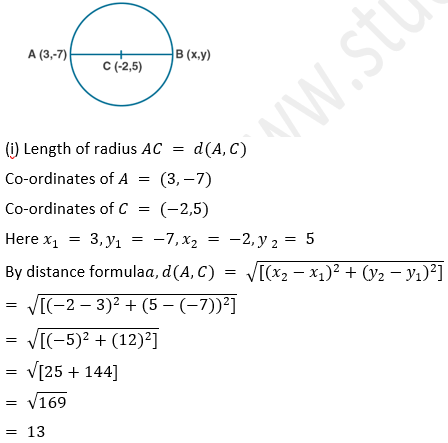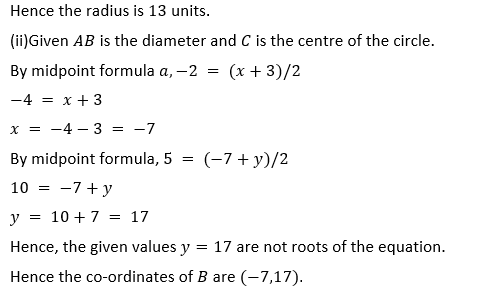14. The line segment joining A(-1,5/3)  the points B (a,5) is divided in the ratio 1∶ 3 at P, the point where the line segment AB intersects y-axis. Calculate
(i) the value of a
(ii) the co-ordinates of P.
Solution:
We have given that:
(i) Let P(x,y) divides the line segment joining the points A(-1,5/3),B(a,5) in the ratio 1:3,
Here m:n = 1:3
x = -1 ,y1  = 5/3 ,x2  = a,y2  = 5
By Section formula x = (mx2+nx1)/(m+n)
x = (1×a+3×-1)/(1+3)
x = (a-3)/4
x = (a-3)/4 …..(i)
By Section formula y = (my2+ny1)/(m+n)
y = (1×5+3×5/3)/(3+1)
y = (5+5)/4
y = 10/4
y = 5/2 ….(ii)
Given P meets Y axis. So its x co-ordinate will be zero.
i.e, (a-3)/4 = 0
a-3 = 0
a = 3
(ii) x = (a-3)/4 [From (i)]
Substitute a = 3 in above equation.
x = (3-3)/4 = 0
y = 5/2 [From (ii)]
Hence, the given values y = 5/2  are not roots of the equation.
Hence the co-ordinates of P are (0,5/2).

15. The point P ( – 4,1) divides the line segment joining the points A (2,– 2) and B in the ratio of 3∶ 5. Find the point B.
Solution:
We have given that:
Let the co-ordinates of B be (x2,y2).
Given co-ordinates of A = (2,-2)
Co-ordinates of P = (-4,1)
Ratio m:n = 3:5
x  = 2,y1  = -2,x = -4,y = 1
P divides AB in the ratio 3:5
By Section formula x = (mx2+nx1)/(m+n)
-4 = (3×x2+5×2)/(3+5)
-4 = (3x2+10)/8
-32 = 3x2+10
3x2  = -32-10 = -42
x2  = -42/3 = -14
By Section formula y = (my2+ny1)/(m+n)
1= (3×y2+5×-2)/(3+5)
1 = (3y2-10)/8
8 = 3y2-10
3y_2  = 8+10 = 18
y = 18/3 = 6
Hence the co-ordinates of B are (-14,6).

16. (i) In what ratio does the point (5,4) divide the line segment joining the points (2,1) and (7 ,6) ?
(ii) In what ratio does the point ( – 4,b) divide the line segment joining the points P (2,– 2),Q ( – 14,6) ? Hence find the value of b.
Solution:
We have given that:
(i) Let the ratio that the point (5,4) divide the line segment joining the points (2,1) and (7,6) be m:n,
Here x1  = 2 ,y1  = 1 ,x2  = 7,y2  = 6,x = 5,y = 4
By Section formula x = (mx2+nx1)/(m+n)
5 = (m×7+n×2)/(m+n)
5 = (7m+2n)/(m+n)
5(m+n) = 7m+2n
5m+5n = 7m+2n
5m-7m = 2n-5n
-2m = -3n
m/n = -3/-2 = 3/2
Hence, the given values m/n = -3/-2 are not roots of the equation.
Hence the ratio m:n is 3:2.

(ii) Let the ratio that the point (-4,b) divide the line segment joining the points (2,-2) and (-14,6) be m:n,
Here x = 2 ,y1  = -2 ,x2  = -14,y2  = 6,x = -4,y = b
By Section formula x = (mx2+nx1)/(m+n)
-4 = (m×-14+n×2)/(m+n)
-4= (-14m+2n)/(m+n)
-4(m+n) = -14m+2n
-4m-4n = -14m+2n
-4m+14m = 2n+4n
10m = 6n
m/n = 6/10 = 3/5
Hence the ratio m:n is 3:5.
By Section formula y = (my2+ny1)/(m+n)
b= (3×6+5×-2)/(3+5)
b = (18-10)/8
b = 8/8
b = 1
Hence, the given values b = 1 are not roots of the equation.
Hence the value of b is 1 and the ratio m:n is 3:5.

17. The line segment joining A (2,3) and B (6,– 5) is intercepted by the x-axis at the point K. Write the ordinate of the point k. Hence, find the ratio in which K divides AB. Also, find the coordinates of the point K.
Solution:
We have given that:
Since the point K is on X axis, its y co-ordinate is zero.
Let the point K be (x,0).
Let the point K divides the line segment joining A(2,3) and B(6,-5) in the ratio m:n.
Here x1  = 2 ,y1  = 3 ,x2  = 6,y2  = -5,y = 0
By Section formula y = (my2+ny1)/(m+n)
0 = (m×-5+n×3)/(m+n)
0 = (-5m+3n)/m+n
-5m+3n = 0
-5m = -3n
m/n = -3/-5 = 3/5
Hence the point K divides the line segment in the ratio 3:5.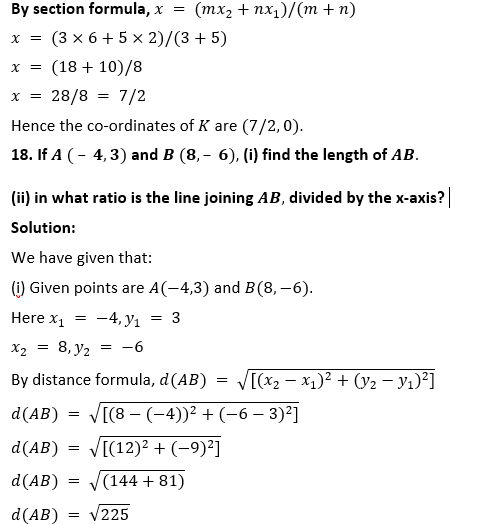d(AB) = 15
Hence the length of AB is 15 units.
(ii)Let m:n be the ratio in which the line AB is divided by the X axis.
Since the line meets X axis, its y co-ordinate is zero.
By Section formula y = (my2+ny1)/(m+n)
0 = (m×-6+n×3)/(m+n)
0 = (-6m+3n)/m+n
-6m+3n = 0
-6m = -3n
m/n = -3/-6 = 3/6 = 1/2
Hence the ratio is 1:2.0 = (-2m+3n)/( m+n)
0 = -2m+3n
2m = 3n
m/n = 3/2
Hence the ration m:n is 3:2.
(ii)Let the line x-y-2 = 0 divide the line segment joining the points (3,-1) and (8,9) in the ratio m:n at the point P(x,y)
Here x1  = 3,y1  = -1
x2  = 8 y2  = 9
By section formula, x = (mx2+nx1)/(m+n)
x = (m×8+n×3)/(m+n)
x = (8m+3n)/( m+n) …. (i)
By Section formula y = (my2+ny1)/(m+n)
y = (m×9+n×-1)/(m+n)
y = (9m-n)/(m+n) …. (ii)
Since the point P(x,y) lies on the line x-y-2 = 0,
eqn (i) and (ii) will satisfy the equation x-y-2 = 0 …(iii)
Substitute (i) and (ii) in (iii)
[(8m+3n)/( m+n)] – [(9m-n)/(m+n)]-2 = 0
[(8m+3n)/( m+n)] – [(9m-n)/(m+n)]-[2(m+n)/(m+n)] = 0
8m+3n-(9m-n)-2(m+n) = 0
8m+3n-9m+n-2m-2n = 0
-3m+2n = 0
-3m = -2n
m/n = -2/-3 = 2/3
Hence the ratio m:n is 2:3.
Substitute m and n in (i)
x = (8m+3n)/( m+n)
x = (8×2+3×3)/(2+3)
x = (16+9)/5
x = 25/5 = 5
Substitute m and n in (ii)
y = (9m-n)/(m+n)
y = (9×2-3)/(2+3)
y = (18-3)/5
y = 15/5 = 3
Hence the co-ordinates of P are (5,3).

20. Given a line segment AB joining the points A ( – 4,6) and B (8,– 3). Find:
(i) the ratio in which AB is divided by the y-axis.
(ii) find the coordinates of the point of intersection.
(iii) the length of AB.
Solution:
We have given that:
(i) Let m:n be the ratio in which the line segment joining A (-4,6) and B(8,-3) is divided by the Y axis.
Since the line meets Y axis, its x co-ordinate is zero.
Here x1  = -4,y1  = 6
x2  = 8,y2  = -3
By section formula, x = (mx2+nx1)/(m+n)
0 = (m×8+n×-4)/(m+n)
0 = (8m+-4n)/(m+n)
0 = 8m+-4n
8m = 4n
m/n = 4/8 = 1/2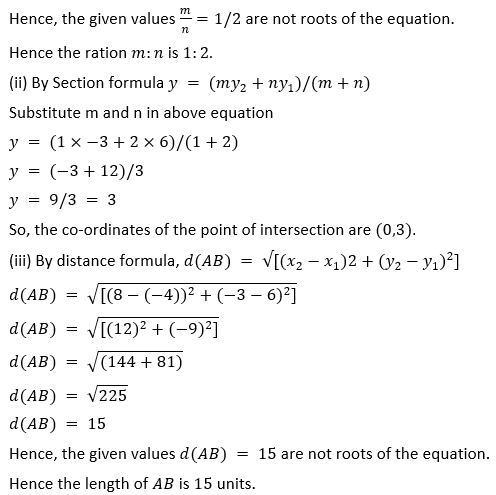21. (i) Write down the co-ordinates of the point P that divides the line joining A ( – 4,1) and B (17,10) in the ratio 1∶ 2.
(ii)Calculate the distance OP where O is the origin.
(iii) In what ratio does the y-axis divide the line AB ?
Solution:
We have given that:
(i)Let P(x,y) divides the line segment joining the points A(-4,1),B(17,10) in the ratio 1:2,
Here x1  = -4,y_1  = 1
x2  = 17,y2  = 10
m:n = 1:2
By section formula, x = (mx2+nx1)/(m+n)
x = (1×17+2×-4)/(1+2)
x = (17+-8)/3
x = 9/3

x = 3
By Section formula y = (my2+ny1)/(m+n)
y = (1×10+2×1)/(1+2)
y = (10+2)/3
y = 12/3 = 4
Hence, the given values y=4 are not roots of the equation.
Hence the co-ordinates of the point P are (3,4).
(ii)Since O is the origin, the co-ordinates of O are (0,0).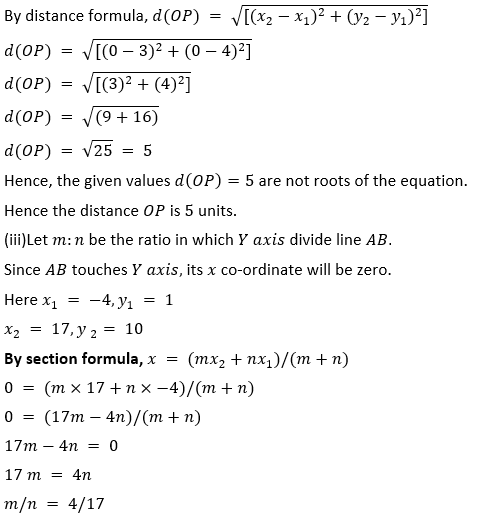Hence, the given values m:n = 4:17 are not roots of the equation.
Hence the ratio in which Y axis divide line AB is 4:17.

22. Calculate the length of the median through the vertex A of the triangle ABC with vertices A (7,– 3),B (5,3) and C (3,– 1).
Solution:
We have given that:
Let M(x,y) be the median of ΔABC through A to BC.
M will be the midpoint of BC.
x1  = 5, y1  = 3
x2  = 3, y2  = -1
By midpoint formula, x = (x1+x2)/2
x = (5+3)/2 = 8/2 = 4
By midpoint formula, y = (y1+y2)/2
y = (3+-1)/2 = 2/2 = 1
Hence the co-ordinates of M are (4,1).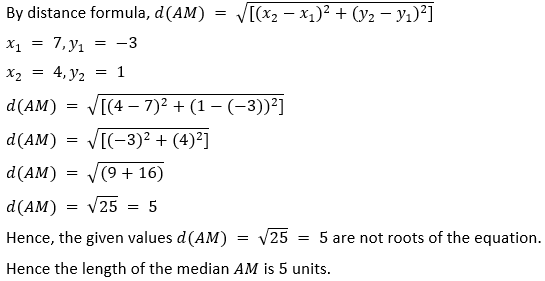23. Three consecutive vertices of a parallelogram ABCD are A (1,2),B (1,0) and C (4,0). Find the fourth vertex D.
Solution:
We have given that:
Let M be the midpoint of the diagonals of the parallelogram ABCD.
Co-ordinate of M will be the midpoint of diagonal AC.
Given points are A(1,2),B(1,0) and C(4,0).
Consider line AC.
x1  = 1, y1= 2
x2  = 4, y2  = 0
By section formula, x = (mx2+nx1)/(m+n)
x = (1+4)/2 = 5/2
By Section formula y = (my2+ny1)/(m+n)
y = (2+0)/2 = 2/2 = 1
Hence the co-ordinates of M are (5/2,1).
M is also the midpoint of diagonal BD.
Consider line BD and M as midpoint.
x1  = 1, y1= 0
x = 5/2,y = 1
By midpoint formula, x = (x1+x2)/2
5/2 = (1+x2)/2
5 = 1+x2
x= 5-1 = 4
By midpoint formula, y = (y1+y2)/2
1 = (0+y2)/2
1 = y2/2
y2  = 2
Hence, the given values y2  = 2 are not roots of the equation.
Hence the co-ordinates of D are (4,2).

24. If the points A ( – 2,– 1),B (1,0),C (p,3) and D (1,q) form a parallelogram ABCD, find the values of p and q.
Solution:
We have given that:Given vertices of the parallelogram are A(-2,-1),B(1,0),C(p,3) and D(1,q).
Let M(x,y) be the midpoint of the diagonals of the parallelogram ABCD.
Diagonals AC and BD bisect each other at M.
When M is the midpoint of AC
By midpoint formula,
x = (-2+p)/2 = (p-2)/2 ..(i)
y = (-1+3)/2 = 2/2 = 1 ..(ii)
When M is the midpoint of BD
By midpoint formula,
x = (1+1)/2 = 2/2 = 1 ..(iii)
y = (q+0)/2 = q/2 ..(iv)
Equating (i) and (iii), we get
(p-2)/2 = 1
p-2 = 2
p = 2+2 = 4Let co-ordinate of C be (x2,y2)
x1  = 3,y_1  = 2
x = 2,y = -5
By midpoint formula, x = (x1+x2)/2
2 = (3+x2)/2
3+x2  = 4
x2  = 4-3 = 1
By midpoint formula, y = (x1+x2)/2
-5 = (2+y2)/2
-10 = 2+y2
y2  = -10-2 = -12
Hence the co-ordinates of the point C are (1,-12).
Consider B-M-D
Let co-ordinate of D be (x2,y2
x1   = -1,y_1  = 0
x = 2,y = -5
By midpoint formula, x = (x1 +x2)/2
2 = (-1+x_2)/2
-1+x_2  = 4
x2  = 4+1 = 5
By midpoint formula, y = (x1 +x2)/2
-5 = (0+y_2)/2
-10 = 0+y_2
y2  = -10
Hence, the given values y2  = -10 are not roots of the equation.
Hence the co-ordinates of the point D are (5,-10).

26. Prove that the points A ( – 5,4),B ( – 1,– 2) and C (5,2) are the vertices of an isosceles right angled triangle. Find the co-ordinates of D so that ABCD is a square.
Solution:
We have given that: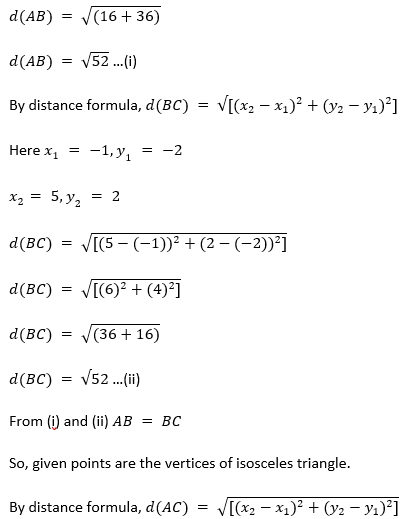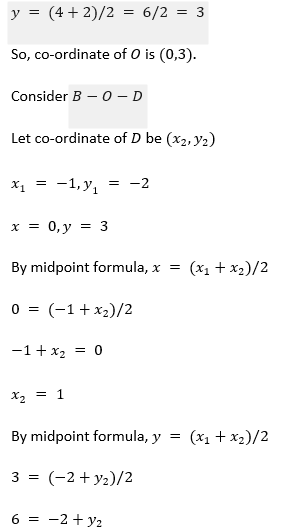y_2  = 6+2 = 8
Hence, the given values y_2=8 are not roots of the equation.
Hence the co-ordinates of the point D are (1,8).

27. Find the third vertex of a triangle if its two vertices are ( – 1,4) and (5,2) and midpoint of one sides is (0,3).
Solution:
We have given that: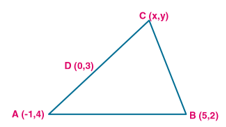Let A (-1,4) and B(5,2) are the vertices of the triangle and let D(0,3) is the midpoint of side AC.
Let co-ordinate of C be (x,y).
Consider D(0,3) as midpoint of AC
By midpoint formula,
(-1+x)/2 = 0
-1 +x = 0
x = 1
By midpoint formula,
(4+y)/2 = 3
4+y = 6
y = 6-4 = 2
So the co-ordinates of C are (1,2).
Consider D(0,3) as midpoint of BC
By midpoint formula,
(5+x)/2 = 0
5 +x = 0
x = -5
By midpoint formula,
(2+y)/2 = 3
2+y = 6
y = 6-2 = 4
So the co-ordinates of C are (-5,4).
Hence the co-ordinates of the point C will be (1,2) or (-5,4).

28. Find the coordinates of the vertices of the triangle the middle points of whose sides are (0,½ ) ,( ½ ,½) and ( ½ ,0).
Solution:
We have given that:Let A(x1,y1),B(x2,y2) and C(x3,y3) be the vertices of the triangle ABC.
Consider AB
By midpoint formula, (x1+x2)/2 = 0
x1+x2  = 0
x1  = -x2 ..(i)
By midpoint formula, (y1+y2)/2 = ½
y1+y2  = 1 …(ii)
Consider AC
By midpoint formula, (x1+x3)/2 = ½
x1+x3  = 1 …(iii)
By midpoint formula, (y1+y3)/2 = 0
y1+y3  = 0
y1  = -y3 …(iv)
Consider BC
By midpoint formula,(x2+x3)/2 = ½
x2+x3  = 1 …(v)
By midpoint formula, (y2+y3)/2 = ½
y2+y3 = 1 …(vi)
Substitute (i) in (iii)
Then (iii) becomes -x2+x3  = 1
Equation (v) x2+x3 = 1
Adding above two equations, we get
2x3  = 2
x3  = 2/2 = 1
Substitute x3  = 1 in (iii), we get x1  = 0
x2  = 0 [From (i)]
So x1  = 0,x2  = 0,x3  = 1
Substitute (iv) in (ii)
Then (ii) becomes -y3+y2 = 1
Equation (vi) y2+y3  = 1
Adding above two equations, we get
2y2  = 2
y2  = 2/2 = 1
Substitute y2  = 1 in (i), we get y1= 0
y3  = 0
So y1  = 0,y2  = 1,y3  = 0
Hence the Co-ordinates of vertices are A(0,0),B(0,1) and C(1,0).

29. Show by section formula that the points (3,– 2),(5,2) and (8,8) are collinear.
Solution:
We have given that:
Let the point B(5,2) divides the line joining A(3,-2) and C(8,8) in the ratio m:n.
Then by section formula, x = (mx2+nx1)/(m+n)
5 = (m×8+n×3)/(m+n)
5 = (8m+3n)/(m+n)
5m+5n = 8m+3n
2n = 3m
m/n = 2/3 …(i)
By section formula, y = (my2+ny1)/(m+n)
2 = (m×8+n×-2)/(m+n)
2 = (8m-2n)/(m+n)
2m+2n = 8m-2n
6m = 4n
m/n = 4/6 = 2/3 …(ii)
Here ratios are same.
So the points are collinear.

30. Find the value of p for which the points ( – 5,1),(1,p) and (4,– 2) are collinear.
Solution:
We have given that:
Let A(-5,1) divides the line joining (1,p) and (4,-2) in the ratio m:n
Then by section formula, x = (mx2+nx1)/(m+n)
-5 = (m×4+n×1)/(m+n)
-5 = (4m+n)/(m+n)
-5m-5n = 4m+n
-9m = 6n
m/n = -9/6 = -2/3 …(i)
By section formula, y = (my2+ny1)/(m+n)
1 = (m×-2+n×p)/(m+n)
1 = (-2m+pn)/(m+n)
m+n = -2m+pn
3m = (p-1)n
m/n = (p-1)/3 ….(ii)
Equating (i) and (ii)
(p-1)/3 = -2/3
p-1 = -2
p = -2+1 = -1
Hence the value of p is -1.

31. A (10,5),B (6,– 3) and C (2,1) are the vertices of triangle ABC. L is the mid point of AB,M is the mid-point of AC. Write down the co-ordinates of L and M. Show that LM = ½ BC.
Solution:
We have given that:
Given points are A(10,5),B(6,-3) and C(2,1).
Let L(x,y) be the midpoint of AB.
Here x1= 10,y1= 5
x2  = 6,y2  = -3
By midpoint formula,x = (x1+x2)/2
x = (10+6)/2 = 16/2 = 8
By midpoint formula, y = (y1+y2 )/2
y = (5-3)/2 = 2/2 = 1
So co-ordinates of L are (8,1).
Let M(x,y) be the midpoint of AC.
Here x1= 10,y1  = 5
x2  = 2,y2   = 1
By midpoint formula, x = (x1+x2)/2
x = (10+2)/2 = 12/2 = 6
By midpoint formula, y = (y1+y2 )/2
y = (5+1)/2 = 6/2 = 3
So co-ordinates of M are (6,3).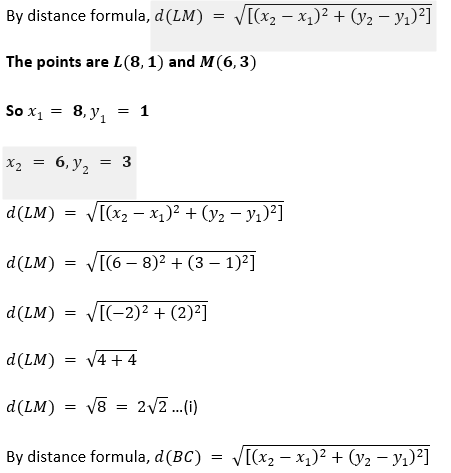32. A (2,5),B ( – 1,2) and C (5,8) are the vertices of a triangle ABC.P and Q are points on AB and AC respectively such that AP∶ PB = AQ∶ QC = 1∶ 2.
(i) Find the co-ordinates of P and Q.
(ii) Show that PQ = 1/3 BC
Solution:
We have given that:(i) Given vertices of the ABC are A(2,5),B(-1,2) and C(5,8).
P and Q are points on AB and AC respectively such that AP:PB = AQ∶QC = 1:2.
P(x,y) divides AB in the ratio 1:2.
x1= 2,y1  = 5
x2  = -1,y2  = 2
m:n = 1:2
By section formula, x = (mx2+nx1)/(m+n)
x = (1×-1+2×2)/(1+2)
x = (-1+4)/(3)
x = 3/3 = 1
By section formula, y = (my2+ny1)/(m+n)
y = (1×2+2×5)/(1+2)
y = (2+10)/(3)
y = 12/3 = 4
Co-ordinates of P are (1,4).
Q(x,y) divides AC in the ratio 1:2.
x1= 2,y1  = 5
x2  = 5,y2  = 8
m:n = 1:2
By section formula, x = (mx2+nx1)/(m+n)
x = (1×5+2×2)/(1+2)
x = (5+4)/(3)
x = 9/3 = 3
By section formula, y = (my2+ny1)/(m+n)
y = (1×8+2×5)/(1+2)
y = (8+10)/(3)
y = 18/3 = 6
Co-ordinates of Q are (3,6).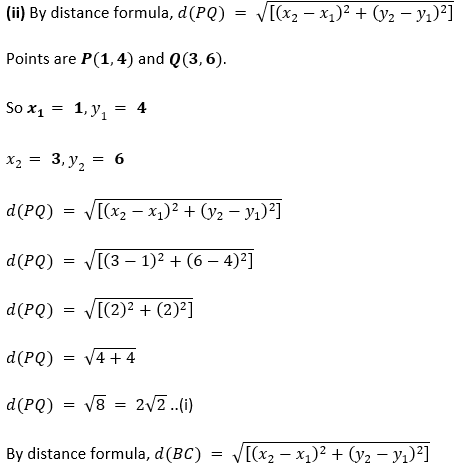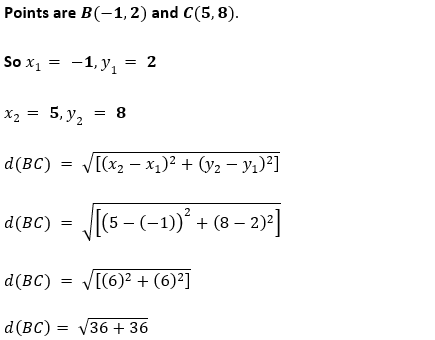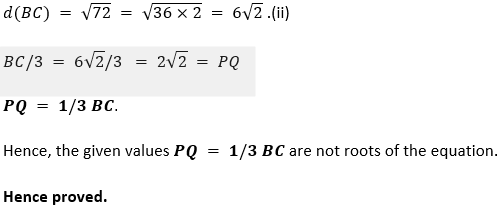33. The mid-point of the line segment AB shown in the adjoining diagram is (4,– 3). Write down the co-ordinates of A and B.Solution:
Let P(4,-3) be the midpoint of line joining the points A and B.
Since A lies on X axis, its co-ordinates are (x2,0)
Since B lies on Y axis, its co-ordinates are (0,y1)
By midpoint formula, x = (x1+x2)/2
4 = (0+x2)/2
x2  = 4×2 = 8
By midpoint formula, y = (y1+y2)/2
-3 = (y1+0)/2
y1= -3×2 = -6
Hence, the given values y1=-6 are not roots of the equation.
Hence the co-ordinates of A and B are (8,0)and (0,-6) respectively.

34. Find the co-ordinates of the centroid of a triangle whose vertices are A ( – 1,3),B(1,– 1) and C (5,1)
Solution:
We have given that:
Given vertices of the triangle are A(-1,3),B(1,-1) and C(5,1)
Co-ordinates of the centroid of a triangle, whose vertices are (x1,y1),(x2,y2) and (x3,y3) are
[(x1+ x2+ x3)/3,(y1+ y2+ y3)/3]
(x1,y1) = (-1,3)
(x2,y2) = (1,-1)
( x3,y3) = (5,1)
[(x1+ x2+ x3)/3 = (-1+1+5)/3 = 5/3
[(y1+ y2+ y3)/3] = (3-1+1)/3 = 3/3 = 1
Hence the co-ordinates of centroid are (5/3,1).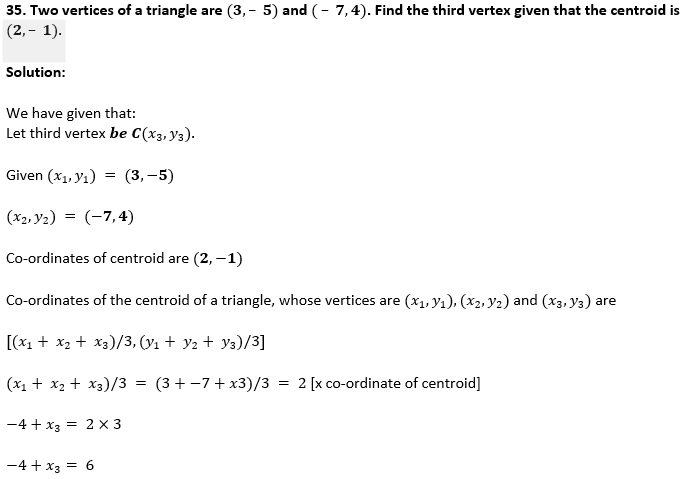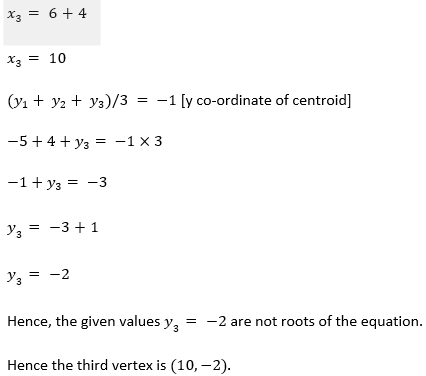36. The vertices of a triangle are A ( – 5,3),B (p,– 1) and C (6,q). Find the values of p and q if the centroid of the triangle ABC is the point (1,– 1).
Solution:
We have given that:
Given vertices of the triangle are A(-5,3),B(p,-1) and C(6,q).
Co-ordinates of centroid are (1,-1).
Co-ordinates of the centroid of a triangle, whose vertices are (x1,y1),(x2,y2) and (x3,y3) are
[(x1+ x2+ x3)/3,(y1+ y2+ y3)/3]
(x1,y1) = (-5,3)
(x2,y2) = (p,-1)
( x3,y3) = (6,q)
x co-ordinate of centroid, [(x1+ x2+ x3)/3 = (-5+p+6)/3 = 1
p+1 = 3
p = 3-1
p = 2
y co-ordinate of centroid, (y1+ y2+ y3)/3 = (3-1+q)/3 = -1
2+q = 3×-1
2+q = -3
q = -3-2
q = -5
Hence the value of p and q are 2 and -5 respectively.